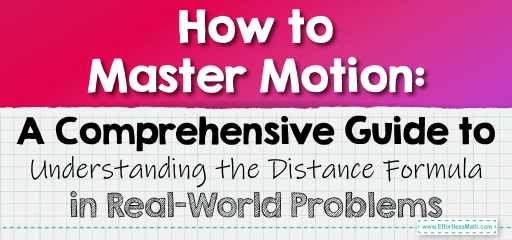# How to Master Motion: A Comprehensive Guide to Understanding the Distance Formula in Real-World Problems

Understanding motion and work problems, especially those involving the formula $$d=rt$$ (where $$d$$ stands for distance, $$r$$ for rate, and $$t$$ for time), can be simplified through a step-by-step guide:## A step-by-step guide to Master Motion

### Step 1: Understanding the Formula

• Formula: $$d=rt$$.
• Distance ($$d$$): This represents the total distance traveled.
• Rate ($$r$$): This is the speed or velocity at which an object moves. It’s expressed as a distance per unit of time (like miles per hour or kilometers per hour).
• Time ($$t$$): The duration for which the object has been moving.

### Step 2: Consistency in Units

• Importance of Consistent Units: It’s crucial to ensure that the units of rate and time match. For example, if the rate is in miles per hour, time should be in hours, not minutes.
• Converting Units: If units do not match, convert them. For instance, if time is given in minutes and rate in hours, convert time to hours by dividing by $$60$$.

### Step 3: Solving for Any Variable

• Solving for Distance ($$d$$): If rate and time are known, multiply them to find the distance.
• Solving for Rate ($$r$$): If distance and time are known, divide the distance by time to find the rate.
• Solving for Time ($$t$$): If distance and rate are known, divide the distance by rate to find the time.

### Step 4: Application in Problems

• Problem Analysis: Identify what is being asked, what is given, and what is unknown.
• Assign Variables: Assign the given values to their respective variables in the formula.
• Solve: Perform the necessary calculations to find the unknown value.

### Step 5: Common Mistakes to Avoid

• Not Converting Units: One of the most common mistakes is not converting units to ensure they are consistent.
• Ignoring Decimal Places: Be precise with calculations, especially when dealing with decimals.
• Misreading the Problem: Carefully read what is asked to correctly identify the known and unknown variables.

### Step 6: Practice and Application

• Practice Problems: Regularly solve different types of motion problems to get a better grasp of the concept.
• Real-Life Application: Apply these concepts to everyday situations like calculating travel time for a trip or the speed of a vehicle.

By following these steps and practicing regularly, understanding motion and work problems, especially those related to the distance formula $$d=rt$$, becomes more manageable and intuitive.

### Examples

Example 1:

A car travels at a speed of $$50$$ miles per hour. How far will it travel in $$3$$ hours?

Solution:

$$Distance = Rate × Time = 50 \ \frac{miles}{hour} × 3 \ hours = 150 \ miles$$.

Example 2:

A cyclist covers a distance of $$90$$ kilometers in $$3$$ hours. What is the cyclist’s average speed?

Solution:

$$Speed = Distance ÷ Time = 90 \ km ÷ 3 \ hours = 30 \frac{km}{hour}$$.

### What people say about "How to Master Motion: A Comprehensive Guide to Understanding the Distance Formula in Real-World Problems - Effortless Math: We Help Students Learn to LOVE Mathematics"?

No one replied yet.

X
30% OFF

Limited time only!

Save Over 30%

SAVE $5 It was$16.99 now it is \$11.99